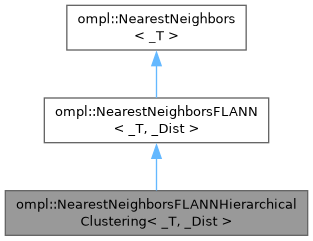ompl::NearestNeighborsFLANNHierarchicalClustering< _T, _Dist > Class Template Reference
Inheritance diagram for ompl::NearestNeighborsFLANNHierarchicalClustering< _T, _Dist >:[legend]Public Types inherited from ompl::NearestNeighbors< _T >
using DistanceFunction = std::function< double(const _T &, const _T &)>
The definition of a distance function.Public Member Functions inherited from ompl::NearestNeighborsFLANN< _T, FLANNDistance< _T > >
NearestNeighborsFLANN (std::shared_ptr< flann::IndexParams > params)

void clear () override
Clear the datastructure.

bool reportsSortedResults () const override
Return true if the solutions reported by this data structure are sorted, when calling nearestK / nearestR.

void setDistanceFunction (const typename NearestNeighbors< _T >::DistanceFunction &distFun) override

void add (const _T &data) override
Add an element to the datastructure.

void add (const std::vector< _T > &data) override

bool remove (const _T &data) override
Remove an element from the datastructure.

_T nearest (const _T &data) const override
Get the nearest neighbor of a point.

void nearestK (const _T &data, std::size_t k, std::vector< _T > &nbh) const override
Return the k nearest neighbors in sorted order if searchParams_.sorted==true (the default)

void nearestR (const _T &data, double radius, std::vector< _T > &nbh) const override
Return the nearest neighbors within distance radius in sorted order if searchParams_.sorted==true (the default)

std::size_t size () const override
Get the number of elements in the datastructure.

void list (std::vector< _T > &data) const override
Get all the elements in the datastructure.

virtual void setIndexParams (const std::shared_ptr< flann::IndexParams > &params)
Set the FLANN index parameters. More...

virtual const std::shared_ptr< flann::IndexParams > & getIndexParams () const
Get the FLANN parameters used to build the current index.

virtual void setSearchParams (const flann::SearchParams &searchParams)
Set the FLANN parameters to be used during nearest neighbor searches.

flann::SearchParams & getSearchParams ()
Get the FLANN parameters used during nearest neighbor searches.

const flann::SearchParams & getSearchParams () const
Get the FLANN parameters used during nearest neighbor searches.

unsigned int getContainerSize () constPublic Member Functions inherited from ompl::NearestNeighbors< _T >
virtual void setDistanceFunction (const DistanceFunction &distFun)
Set the distance function to use.

const DistanceFunctiongetDistanceFunction () const
Get the distance function used.

virtual bool reportsSortedResults () const =0
Return true if the solutions reported by this data structure are sorted, when calling nearestK / nearestR.

virtual std::size_t size () const =0
Get the number of elements in the datastructure.Protected Member Functions inherited from ompl::NearestNeighborsFLANN< _T, FLANNDistance< _T > >
void createIndex (const flann::Matrix< _T > &mat)
Internal function to construct nearest neighbor data structure with initial elements stored in mat.

void createIndex (const flann::Matrix< double > &mat)

void rebuildIndex (unsigned int capacity=0)
Rebuild the nearest neighbor data structure (necessary when changing the distance function or index parameters).Protected Attributes inherited from ompl::NearestNeighborsFLANN< _T, FLANNDistance< _T > >
std::vector< _T > data_
vector of data stored in FLANN's index. FLANN only indexes references, so we need store the original data.

flann::Index< FLANNDistance< _T > > * index_
The FLANN index (the actual index type depends on params_).

std::shared_ptr< flann::IndexParams > params_
The FLANN index parameters. This contains both the type of index and the parameters for that type.

flann::SearchParams searchParams_
The parameters used to seach for nearest neighbors.

unsigned int dimension_
If each element has an array-like structure that is exposed to FLANN, then the dimension_ needs to be set to the length of this array.Protected Attributes inherited from ompl::NearestNeighbors< _T >
DistanceFunction distFun_
The used distance function.

## Detailed Description

### template<typename _T, typename _Dist = FLANNDistance<_T>> class ompl::NearestNeighborsFLANNHierarchicalClustering< _T, _Dist >

Definition at line 374 of file NearestNeighborsFLANN.h.

The documentation for this class was generated from the following file: